# STATISTICAL ANALYSIS WITH EXCEL Purpose of a Spreadsheet

• Slides: 26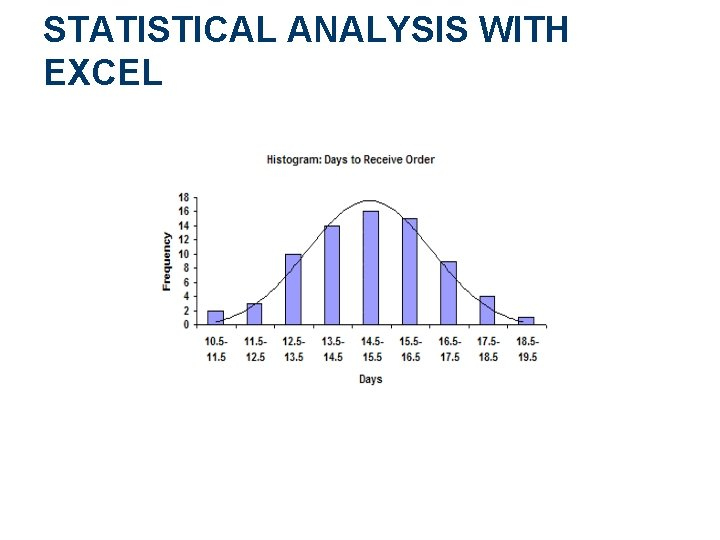STATISTICAL ANALYSIS WITH EXCEL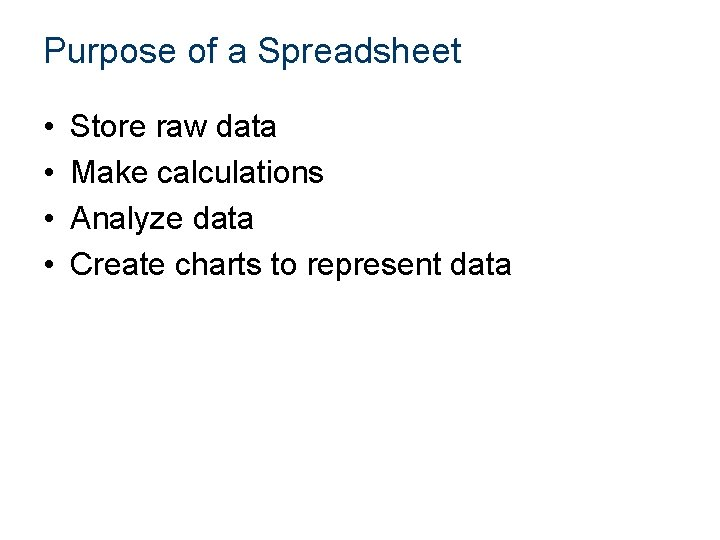Purpose of a Spreadsheet • • Store raw data Make calculations Analyze data Create charts to represent data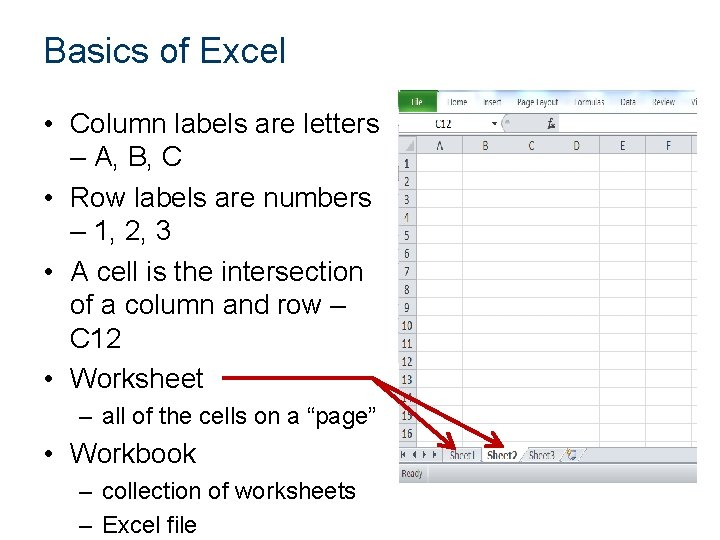Basics of Excel • Column labels are letters – A, B, C • Row labels are numbers – 1, 2, 3 • A cell is the intersection of a column and row – C 12 • Worksheet – all of the cells on a “page” • Workbook – collection of worksheets – Excel file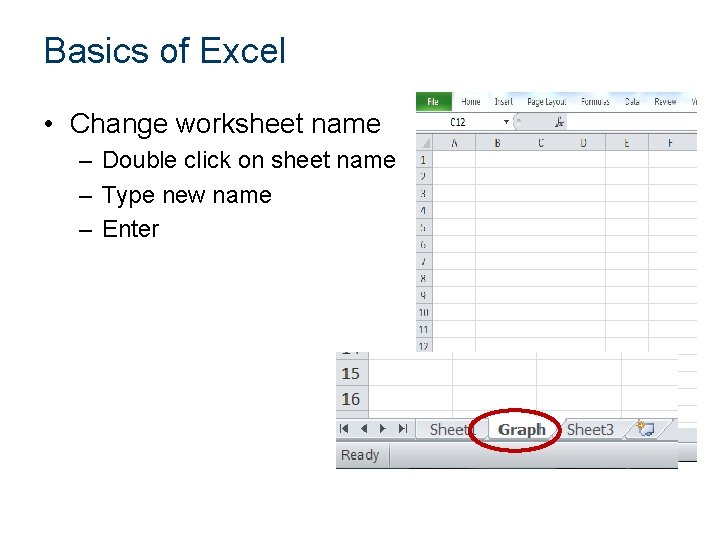Basics of Excel • Change worksheet name – Double click on sheet name – Type new name – Enter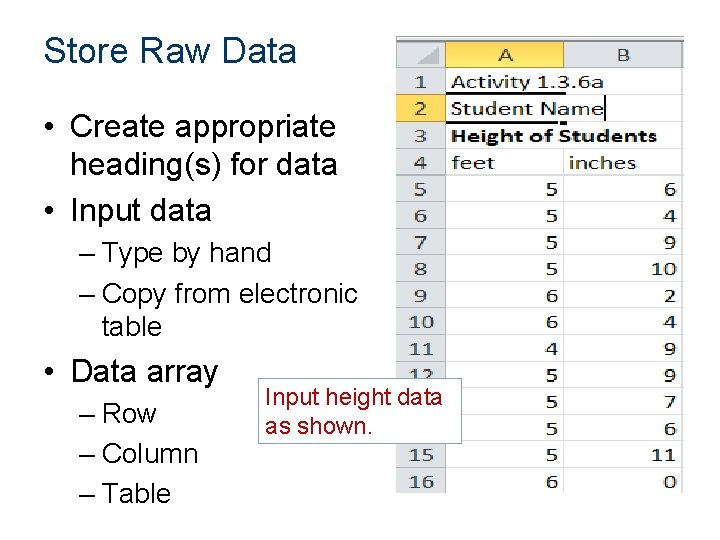Store Raw Data • Create appropriate heading(s) for data • Input data – Type by hand – Copy from electronic table • Data array – Row – Column – Table Input height data as shown.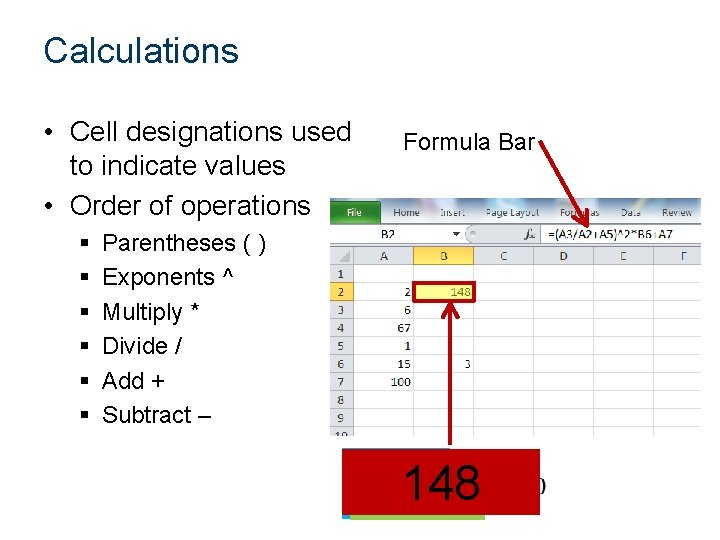Calculations • Cell designations used to indicate values • Order of operations § § § Formula Bar Parentheses ( ) Exponents ^ Multiply * Divide / Add + Subtract – 148 48 3 4 16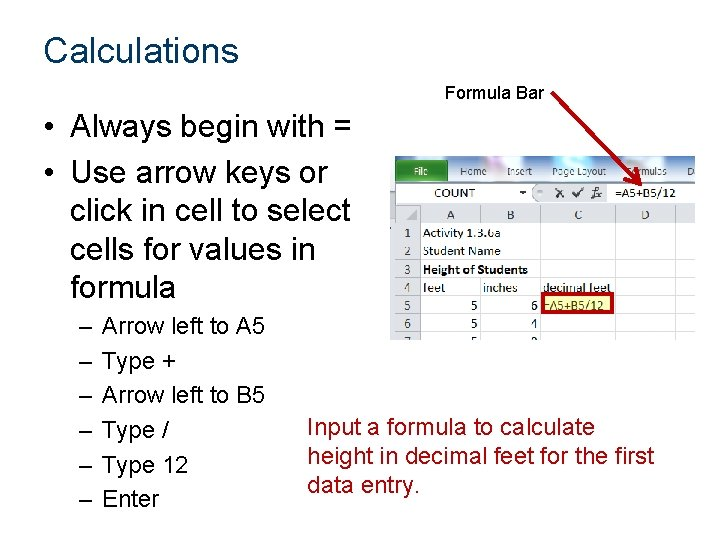Calculations Formula Bar • Always begin with = • Use arrow keys or click in cell to select cells for values in formula – – – Arrow left to A 5 Type + Arrow left to B 5 Type / Type 12 Enter Input a formula to calculate height in decimal feet for the first data entry.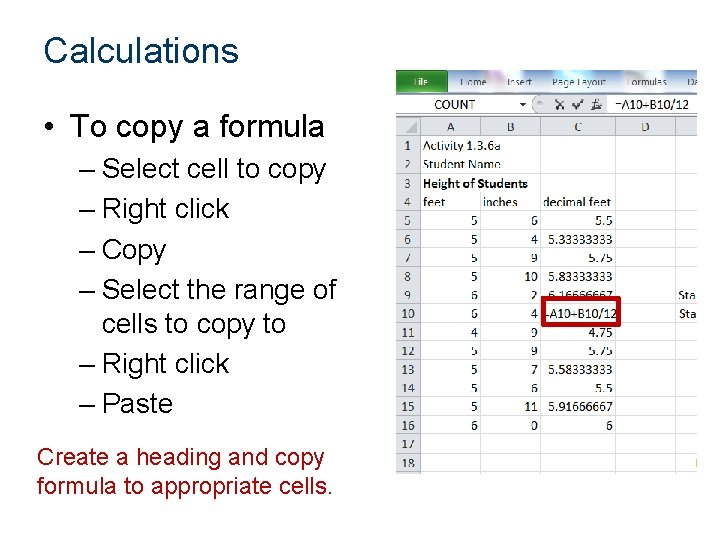Calculations • To copy a formula – Select cell to copy – Right click – Copy – Select the range of cells to copy to – Right click – Paste Create a heading and copy formula to appropriate cells.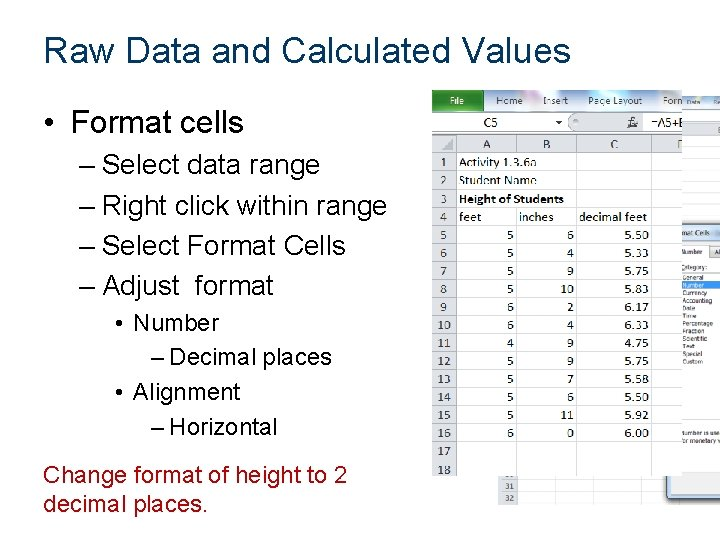Raw Data and Calculated Values • Format cells – Select data range – Right click within range – Select Format Cells – Adjust format • Number – Decimal places • Alignment – Horizontal Change format of height to 2 decimal places.Calculations • Functions – Defined mathematical calculation Function Excel command Addition of numbers Mean Median Standard Deviation (population) Mode (unimodal) Minimum Maximum SUM AVERAGE MEDIAN STDEV. P MODE. SNGL MIN MAX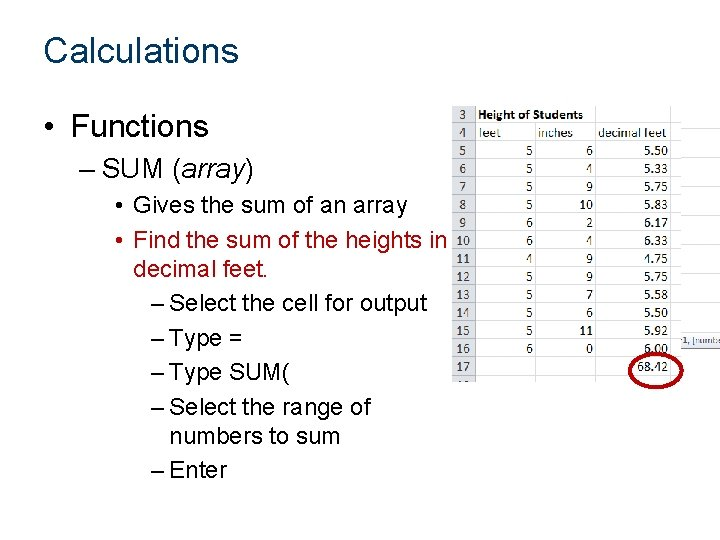Calculations • Functions – SUM (array) • Gives the sum of an array • Find the sum of the heights in decimal feet. – Select the cell for output – Type = – Type SUM( – Select the range of numbers to sum – Enter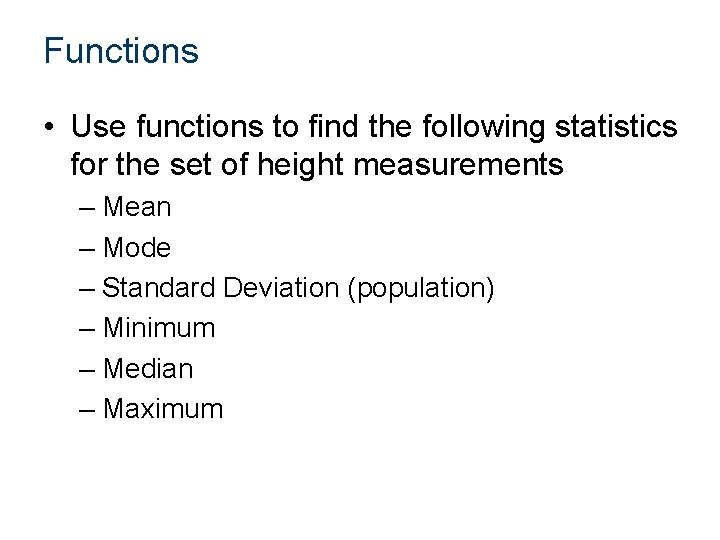Functions • Use functions to find the following statistics for the set of height measurements – Mean – Mode – Standard Deviation (population) – Minimum – Median – Maximum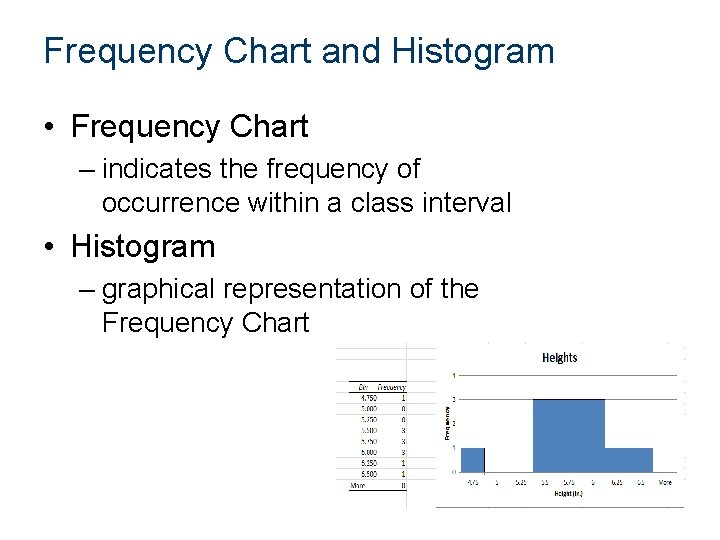Frequency Chart and Histogram • Frequency Chart – indicates the frequency of occurrence within a class interval • Histogram – graphical representation of the Frequency Chart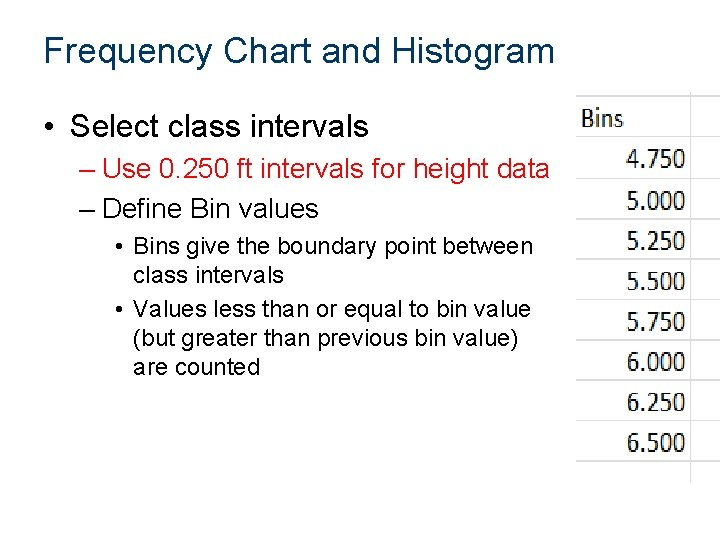Frequency Chart and Histogram • Select class intervals – Use 0. 250 ft intervals for height data – Define Bin values • Bins give the boundary point between class intervals • Values less than or equal to bin value (but greater than previous bin value) are countedFrequency Chart and Histogram • Select the Data Analysis Tool – Data tab > Analysis panel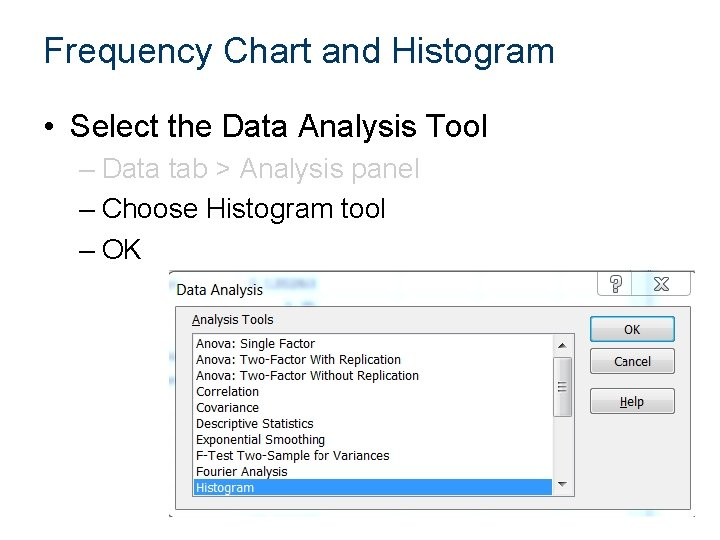Frequency Chart and Histogram • Select the Data Analysis Tool – Data tab > Analysis panel – Choose Histogram tool – OKFrequency Chart and Histogram • Select the Data Analysis Tool – Data tab > Analysis panel – Choose Histogram tool – OK – Select Input Range – Select Bin Range – Choose Output Range • Select a cell on the worksheet – Check Chart Output – OK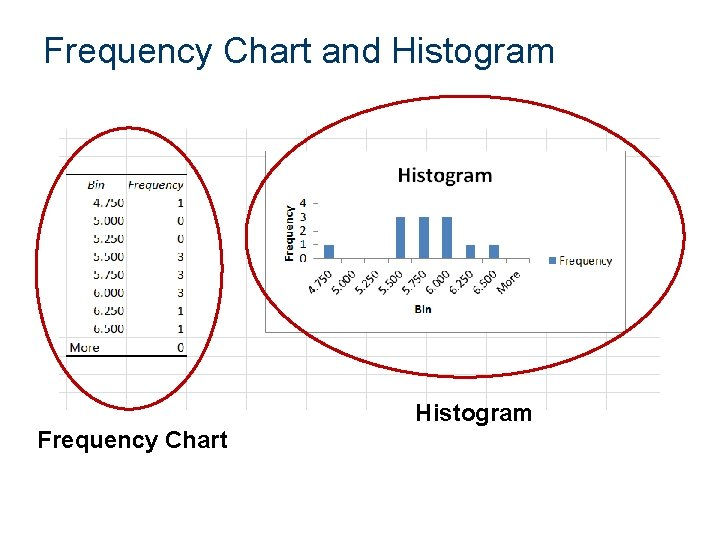Frequency Chart and Histogram Frequency ChartHistogram • Format Histogram – Rotate height text • Select height numbers on chart • Double click on selection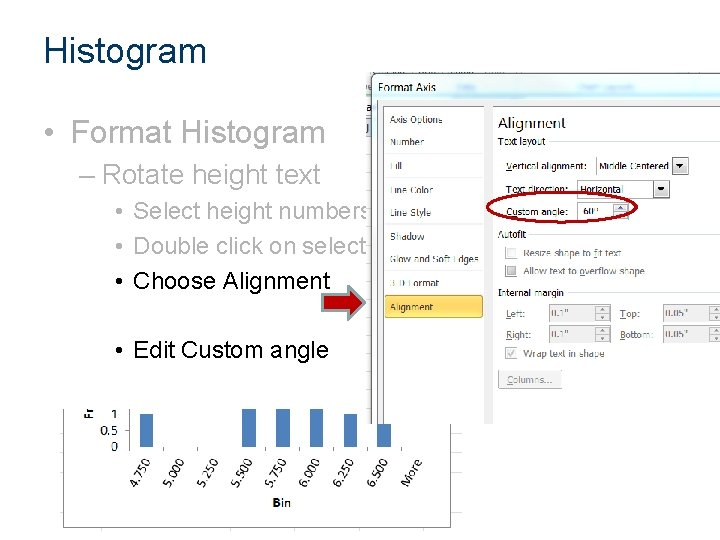Histogram • Format Histogram – Rotate height text • Select height numbers on chart • Double click on selection • Choose Alignment • Edit Custom angle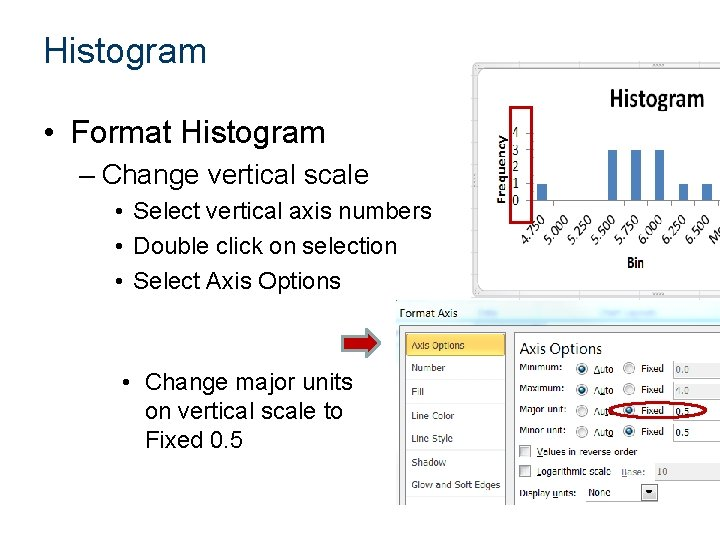Histogram • Format Histogram – Change vertical scale • Select vertical axis numbers • Double click on selection • Select Axis Options • Change major units on vertical scale to Fixed 0. 5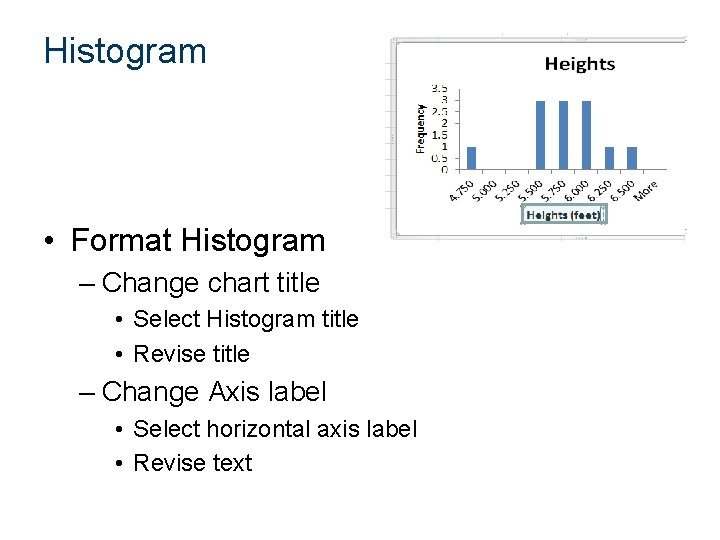Histogram • Format Histogram – Change chart title • Select Histogram title • Revise title – Change Axis label • Select horizontal axis label • Revise text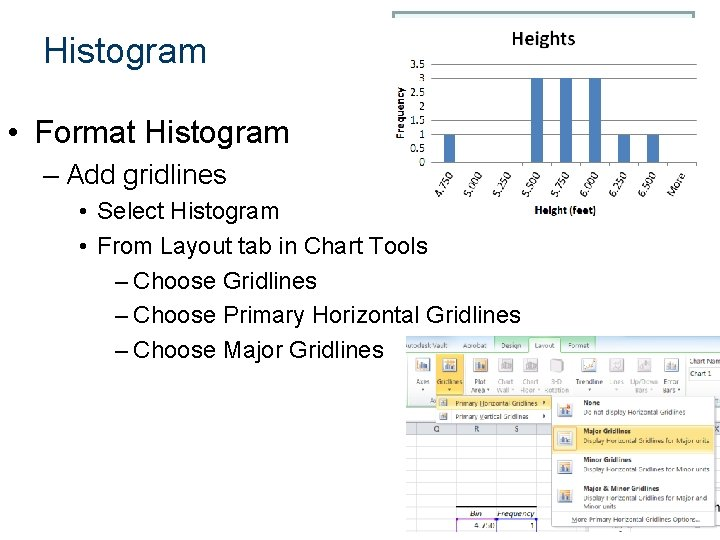Histogram • Format Histogram – Add gridlines • Select Histogram • From Layout tab in Chart Tools – Choose Gridlines – Choose Primary Horizontal Gridlines – Choose Major Gridlines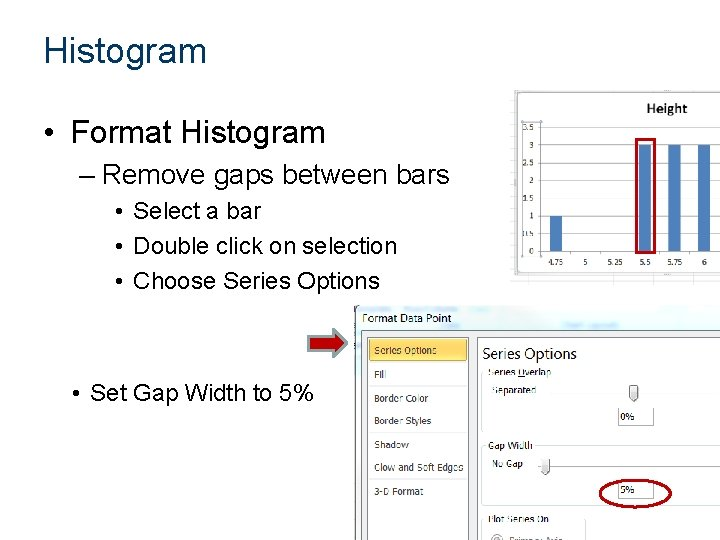Histogram • Format Histogram – Remove gaps between bars • Select a bar • Double click on selection • Choose Series Options • Set Gap Width to 5%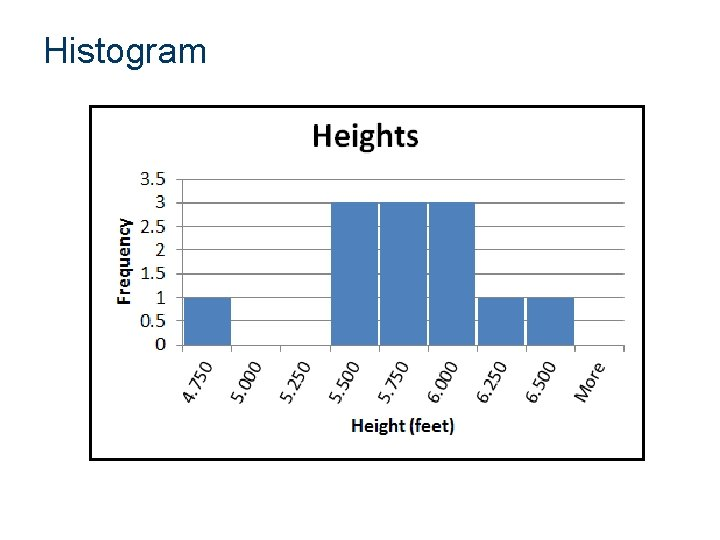Histogram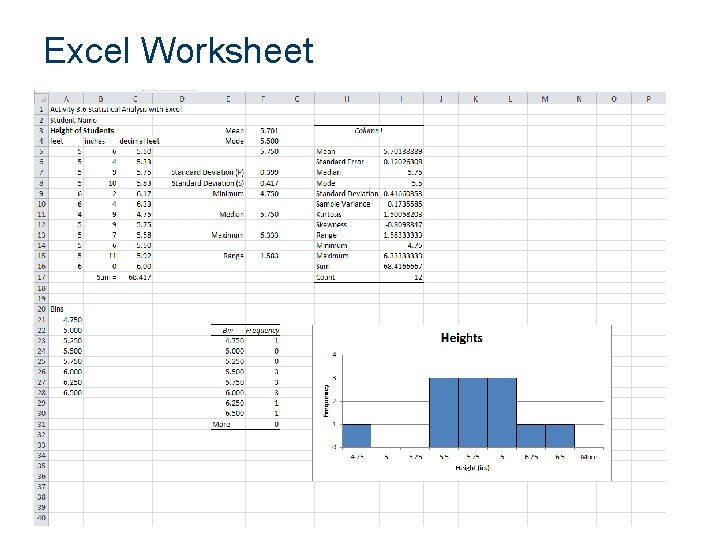Excel Worksheet